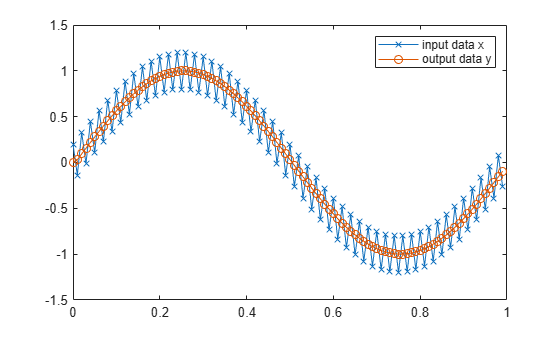Documentation

# subsasgn

Subscripted assignment

## Syntax

```a(I) = b a(I,J) = b a(I,:) = b a(:,I) = b a(I,J,K,...) = b a = subsasgn(a,S,b) ```

## Description

`a(I) = b` assigns the values of `b` into the elements of `a` specified by the subscript vector `I`. `b` must have the same number of elements as `I` or be a scalar value.

`a(I,J) = b` assigns the values of `b` into the elements of the rectangular submatrix of `a` specified by the subscript vectors `I` and `J`. `b` must have `LENGTH(I)` rows and `LENGTH(J)` columns.

A colon used as a subscript, as in `a(I,:) = b` or ```a(:,I) = b``` indicates the entire column or row.

For multidimensional arrays, `a(I,J,K,...) = b` assigns `b` to the specified elements of `a`. `b` must be `length(I)`-by-`length(J)`-by-`length(K)`-... or be shiftable to that size by adding or removing singleton dimensions.

`a = subsasgn(a,S,b)` is called for the syntax `a(i)=b`, `a{i}=b`, or `a.i=b` when `a` is an object. `S` is a structure array with the following fields:

• type — One of the following: `'()'`, `'{}'`, or `'.'` specifying the subscript type

• subs — Cell array or character vector containing the actual subscripts

For instance, the syntax `a(1:2,:) = b` calls `a=subsasgn(a,S,b)` where `S` is a 1-by-1 structure with `S.type='()'` and ```S.subs = {1:2,':'}```. A colon used as a subscript is passed as `':'`.

You can use fixed-point assignment, for example ```a(:) = b```, to cast a value with one `numerictype` object into another `numerictype` object. This subscripted assignment statement assigns the value of `b` into `a` while keeping the `numerictype` object of `a`. Subscripted assignment works the same way for integer data types.

## Examples

collapse all

For `fi` objects `a` and `b`, there is a difference between

`a = b`

and

`a(:) = b`

In the first case, `a = b` replaces `a` with `b` while `a` assumes the value, `numerictype` object and `fimath` object associated with `b`. In the second case, `a(:) = b` assigns the value of `b` into `a` while keeping the `numerictype` object of `a`. You can use this to cast a value with one `numerictype` object into another `numerictype` object.

For example, cast a 16-bit number into an 8-bit number.

`a = fi(0, 1, 8, 7)`
```a = 0 DataTypeMode: Fixed-point: binary point scaling Signedness: Signed WordLength: 8 FractionLength: 7 ```
`b = fi(pi/4, 1, 16, 15)`
```b = 0.7854 DataTypeMode: Fixed-point: binary point scaling Signedness: Signed WordLength: 16 FractionLength: 15 ```
`a(:) = b`
```a = 0.7891 DataTypeMode: Fixed-point: binary point scaling Signedness: Signed WordLength: 8 FractionLength: 7 ```

This example defines a variable `acc` to emulate a 40-bit accumulator of a DSP. The products and sums in this example are assigned into the accumulator using the syntax `acc(1)=...` Assigning values into the accumulator is like storing a value in a register. To begin, turn the logging mode on and define the variables. In this example, `n` is the number of points in the input data `x` and output data `y`, and `t` represents time. The remaining variables are all defined as `fi` objects. The input data `x` is a high-frequency sinusoid added to a low-frequency sinusoid.

```fipref('LoggingMode', 'on'); n = 100; t = (0:n-1)/n; x = fi(sin(2*pi*t) + 0.2*cos(2*pi*50*t)); b = fi([.5 .5]); y = fi(zeros(size(x)), numerictype(x)); acc = fi(0.0, true, 40, 30);```

The following loop takes a running average of the input `x` using the coefficients in `b` . Notice that `acc` is assigned into `acc(1)=...` versus using `acc=...`, which would overwrite and change the data type of `acc` .

```for k = 2:n acc(1) = b(1)*x(k); acc(1) = acc + b(2)*x(k-1); y(k) = acc; end```

By averaging every other sample, the loop shown above passes the low-frequency sinusoid through and attenuates the high-frequency sinusoid.

```plot(t,x,'x-',t,y,'o-') legend('input data x','output data y')```The log report shows the minimum and maximum logged values and ranges of the variables used. Because `acc` is assigned into, rather than overwritten, these logs reflect the accumulated minimum and maximum values.

`logreport(x, y, b, acc)`
``` minlog maxlog lowerbound upperbound noverflows nunderflows x -1.200012 1.197998 -2 1.999939 0 0 y -0.9990234 0.9990234 -2 1.999939 0 0 b 0.5 0.5 -1 0.9999695 0 0 acc -0.9990234 0.9989929 -512 512 0 0 ```

Display `acc` to verify that its data type did not change.

`acc`
```acc = -0.0941 DataTypeMode: Fixed-point: binary point scaling Signedness: Signed WordLength: 40 FractionLength: 30 ```

Reset the fipref object to restore its default values.

`reset(fipref)`

## Extended Capabilities

### GPU Code GenerationGenerate CUDA® code for NVIDIA® GPUs using GPU Coder™.